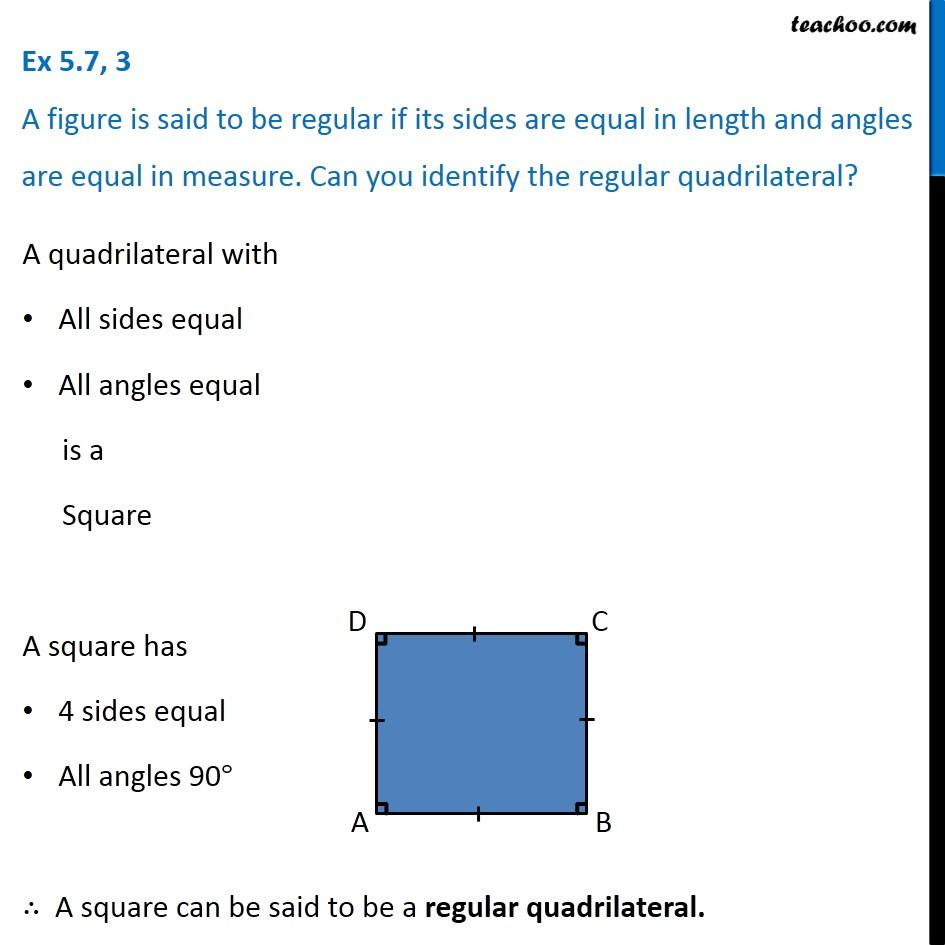1. Chapter 5 Class 6 Understanding Elementary Shapes
2. Serial order wise
3. Ex 5.7

Transcript

Ex 5.7, 3 A figure is said to be regular if its sides are equal in length and angles are equal in measure. Can you identify the regular quadrilateral? A quadrilateral with All sides equal All angles equal is a Square A square has 4 sides equal All angles 90° ∴ A square can be said to be a regular quadrilateral.

Ex 5.7